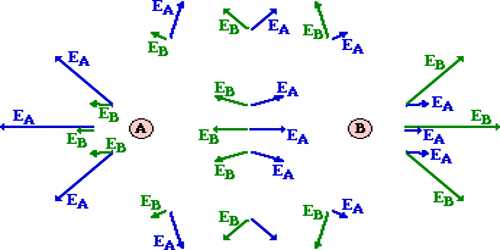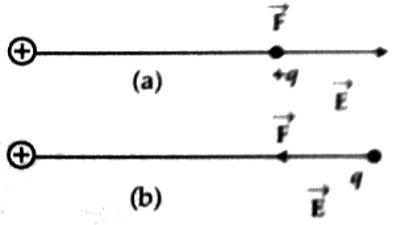# Electric Field Intensity or Electric Intensity

Electric field intensity or Electric intensity

Everywhere in the electric field, its influence is not same. The electric force which a test charge experiences near a charged body is more than at a distance far from it. Again if the charge of a body is more than test charge will experience more force than a body of small charge at the same point. The strength or weakness of the electric field is expressed by a quantity which is called electric intensity. The force acting between a point charge and a test charge at the same point of the electric field is different according to the variation of the magnitude of the test charge, but for a unit test charge, the force remains same. So we can define electric field intensity in the following way.

The electric force acting on a unit charge at a point in the electric field is called electric field intensity. It is also called field intensity.

The electric field is expressed by E. It is a vector quantity.Since the electric field is a vector quantity so it has both magnitude and direction. The direction of E is along the force acting on a positive charge. (Fig (a)], in case of negative charge direction of E is in the opposite direction of F [Fig (b)].

If the force acting on a test charge q0 placed at a point in the electric field is F, then the electric field at that point is,

E = F/q0

then, F = q0E

This equation gives the relation between electric field and electric force.

If the magnitude of the electric force in the electric field is divided by the charge, then the result will give the magnitude of the electric field intensity or electric field.